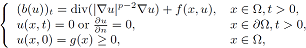##### Analytical Model for Childhood Pneumonia, a Case Study of Kenya

C. G. Ngari, G. P. Pokhariyal, J. K. Koske

Journal of Advances in Mathematics and Computer Science, Page 1-28
DOI: 10.9734/BJMCS/2016/20180

Pneumonia is an infection of the lungs that is caused by bacteria, viruses, fungi, or parasites. For a long time to the best of our knowledge there have not been reliable mathematical model for childhood pneumonia in Kenya. This research study developed a deterministic model based on the Susceptible-Vaccinated-Infected-Treated-Recovered-Susceptible compartment classes. The study used the partial differentiation of control reproduction number (RC)  toinvestigate effects of; environment, efficacy of vaccination drug and treatment. Model analysis indicates the system lie in feasible region, it is bounded, has no backward bifurcation and there exists unique endemic equilibrium point when control reproduction number is greater than unity. Local and global stability of the equilibrium points indicated that control reproduction has to be maintained at less than unity to eradicate the disease. Sensitivity analysis of the control reproduction number indicates that improved vaccination drug’s efficacy, attaining herd immunity, higher treatment rates and lower effects of environment are the best intervention strategies to lower impact of the pneumonia of the children under the age of five years in Kenya.

##### Numerical Solution of Fuzzy Partial Differential Equations by Using Modified Fuzzy Neural Networks

Eman A. Hussian, Mazin H. Suhhiem

Journal of Advances in Mathematics and Computer Science, Page 1-20
DOI: 10.9734/BJMCS/2016/20504

The aim of this work is to present a modified method for finding the numerical solutions of fuzzy partial differential equations by using fuzzy artificial neural networks. Using a fuzzy trial neural solution depending on the fuzzy initial values ​​and the fuzzy boundary conditions of the problem. Using modified fuzzy neural network makes that training points should be selected over an open interval without training the network in the range of first and end points. In fact, This new method based on replacing each element in the training set by a polynomial of first degree. The fuzzy trial solution of fuzzy partial differential equation is written as a sum of two parts. The first part satisfies the fuzzy conditions, it contains no fuzzy adjustable parameters. The second part involves a feed-forward fuzzy neural network containing fuzzy adjustable parameters. In comparison with existing similar fuzzy neural networks, the proposed method provides solutions with high accuracy. Finally, we illustrate our approach by two problems.

##### RETRACTED: A New Approach to Output Control of Smart Beams under Axial and Transverse Loads

Mark A. Pinsky, Steve Koblik

Journal of Advances in Mathematics and Computer Science, Page 1-15
DOI: 10.9734/BJMCS/2016/20644

A problem of suppressing vibrations of elastic structures through controlling their finitedimensional approximations was addressed in various publications which primarily utilize collocated sensors and actuators. This engineering approach, however, raised concerns that a control designed for a finite dimensional model could excite or destabilize its abandoned infinitedimensional complement, which reflects in so known spillover phenomenon. This paper developed a novel design of an output controller, which eliminates spillover in control and marginalizes its contribution to observer design. This consequently leads to an efficient control of beam vibrations, which can be attained by non-collocated sensors and actuators located nearly anywhere on the beam. We show in simulations that the proposed methodology leads to stabilization of postcritical buckling and an efficient reduction of the beam’s resonance vibrations enforced by loads comprised of an axial force and unknown transverse bounded disturbances. We infer controllability and observability conditions which pinpoint areas on the beam where locating actuators/sensors should be avoided and estimated the contribution of discarded part of the initial system.

Retraction Notice: After formal procedures editor(s) and publisher have retracted this paper on 29th July-2016. Related policy is available here: http://goo.gl/lI77Nn

##### Bounds for the Blow-up Time and Blow-up Rate Estimates for Nonlinear Parabolic Equations with Dirichlet or Neumann Boundary Conditions

Yuhua Jian, Zuodong Yang

Journal of Advances in Mathematics and Computer Science, Page 1-12
DOI: 10.9734/BJMCS/2016/20736

This paper is concerned with the blow-up phenomena for a type of parabolic equations with
weighted nonlinear sourcewhere Ω ⊂ RN  (N ≥ 3) is a smooth bounded domain. Through constructing some suitable auxiliary functions and using the rst-order di erential inequality technique, we obtain the bounds for the blow-up time and the estimates of the blow-up rate of the solution to the problem.

##### Linear Metrics and Effective Separating Sequences

Zvonko Iljazović

Journal of Advances in Mathematics and Computer Science, Page 1-8
DOI: 10.9734/BJMCS/2016/21852

On the real line equipped with the Euclidean metric every two effective separating sequences which have a common computable point are equivalent. We prove that the same result holds for every linear metric on the real line.

##### The Sum and Product of Chromatic Numbers of Graphs and their Line Graphs

C. Susanth, Sunny Joseph Kalayathankal

Journal of Advances in Mathematics and Computer Science, Page 1-7
DOI: 10.9734/BJMCS/2016/20823

The bounds on the sum and product of chromatic numbers of a graph and its complement are known as Nordhaus-Gaddum inequalities. In this paper, some variations on this result is studies. First, recall their theorem, which gives bounds on the sum and the product of the chromatic number of a graph with that of its complement. We also provide a new characterization of the certain graph classes.

##### The Degree of a p-group G with | G' | = p

Let G be a finite group and δ (G) denote the least n ∈such that G is isomorphic to a subgroup of the symmetric group Sn. The number δ (G) is called the degree of G. This paper is a note on δ (G) for a nite p-group G with derived subgroup of order p. Our method is based on quasi- permutation representations of a finite group.